Courses

# Previous year Questions (2016-20) - Semiconductor devices, Communications systems (Part - 2) Notes | EduRev

## JEE : Previous year Questions (2016-20) - Semiconductor devices, Communications systems (Part - 2) Notes | EduRev

The document Previous year Questions (2016-20) - Semiconductor devices, Communications systems (Part - 2) Notes | EduRev is a part of the JEE Course Physics Class 12.
All you need of JEE at this link: JEE

Q 28.  A carrier wave of peak voltage 14V is used for transmitting a message signal given to achieve a modulation index of 80% will be:    
(1) 22.4V
(2) 7V
(3) 11.2V
(4) 28V
Ans:
(3)
Solution:
Modulation Index-
The ratio of change of amplitude of carrier wave to the amplitude of original carrier wave.
-wherein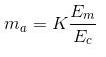modulation index (μ) = Am / Ac
Ac = 14v, μ = 0.8
Am = 14 * 0.8 = 11.2V

Q 29.  In a common emitter amplifier circuit using an n-p-n transistor, the phase difference between the input and the output voltages will be    
(1) 135°
(2) 180°
(3) 45°
(4) 90°
Ans:
(2)
Solution:
In common emitter configuration for n-p-n transistor, phase difference between output and input voltage is 180°.

Q 30. In amplitude modulation, sinusoidal carrier frequency used is denoted by ωc and the signal frequency is denoted by ωm. The bandwidth (Δωm) of the signal is such that Δωm << ωmc. Which of the following frequencies is not contained in the modulated wave?
(1) ωm + ωc
(2) ωc + ωm
(3) ωm
(4) ωc
Ans:
(3)
Solution:
Modulated wave has frequency range.
ωc ± ωm
∴ Since ωc >> ωm
∴ ωm is excluded.

Q 31. A magnetic dipole in a constant magnetic field has:
(1) maximum potential energy when the torque is maximum
(2) zero potential energy when the torque is minimum
(3) zero potential energy when the  torque is maximum
(4) minimum potential energy when the torque is maximum    
Ans:
B
Solution:
PE = – PE cos θ
τ = PE sin θ
τmax when θ = 90°
PE = 0

Q 32. A small circular loop of wire of radius a is located at the centre of a much larger circular wire loop of radius b. The two loops are on the same plane. The outer loop of radius b carries an alternating current I=Io cos (ωt). The emf induced in the smaller inner loop is nearly:    
(1)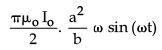(2)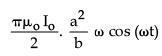(3)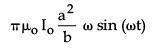(4)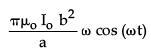Ans:
B
Solution: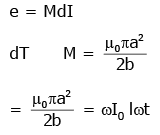Q 33. A 1 kg block attached to a spring vibrates with a frequency of 1 Hz on a frictionless horizontal table. Two springs identical to the original spring are attached in parallel to an 8 kg block placed on the same table. So, the frequency of vibration of the 8 kg block is:    
(1) 1/4Hz
(2)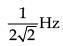(3)  1/2 Hz
(4) 2Hz
Ans:
(2)
Solution: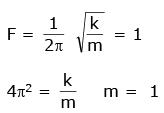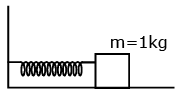k = 4π2
In parallel keq = 2k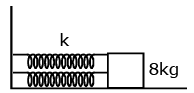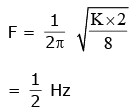Q 34.  A uniform magnetic field B of 0.3 T is along the positive Z-direction. A rectangular loop (abcd) of sides 10 cm × 5 cm carries a current I of 12 A. Out of the following different orientations which one corresponds to stable equilibrium?    
(1)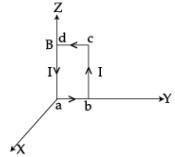(2)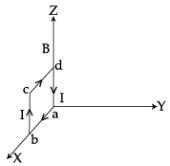(3)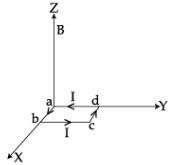(4)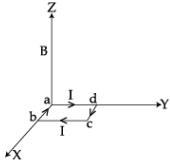Ans:
(4)
Solution:
M = NIA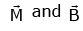are on same direction

Q 35. A steel rail of length 5 m and area of cross-section 40 cm2 is prevented from expanding along its length while the temperature rises by 10C. If coefficient of linear expansion and Young’s modulus of steel are 1.2 × 10−5 K−1 and 2 × 1011 Nm−2 respectively, the force developed in the rail is approximately:    
(1) 2×107 N
(2) 1×105 N
(3) 2×109 N
(4) 3×10−5 N
Ans:
(2)
Solution:
F = yA∝ Δt
= 2 × 1011 × 40 × 10-4 × 1.2 × 10-5 × 10
= 9.6 × 104 = 1 × 105 N

Q 36.  Identify the semiconductor devices whose characteristics are given below, in the order (a), (b), (c), (d):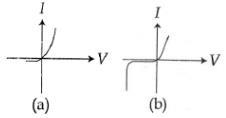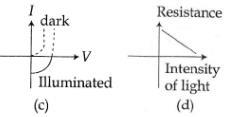(1) Simple diode, Zener diode, Solar cell, Light dependent resistance
(2) Zener diode, Simple diode, Light dependent resistance, Solar cell
(3) Solar cell, Light dependent resistance, Zener diode, Simple diode
(4) Zener diode, Solar cell, Simple diode, Light dependent resistance
Ans:
(1)
Solution:
Graph (a) is for a simple diode.
Graph(b) is showing the V Break down used for zener diode.
Graph (c) is for solar cell which shows cut-off voltage and open circuit current
Graph (d) shows the variation of resistance h and hence current with intensity of light.

Q 37. For a common emitter configuration, if a and b have their usual meanings, the incorrect relationship between a and b is:    
(1)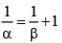(2)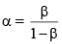(3)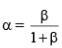(4)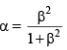Ans:
(2)
Solution:
We know that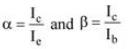Also Ic = Ib + Ic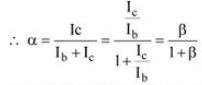Options (1) and (3) are therefore correct.

Q 38. Choose the correct statement :
(1) In amplitude modulation the amplitude of the high frequency carrier wave is made to vary in proportion to the amplitude of the audio signal
(2) In amplitude modulation the frequency of the high frequency carrier wave is made to vary in proportion to the amplitude of the audio signal
(3) In frequency modulation the amplitude of the high frequency carrier wave is made to vary in proportion to the amplitude of the audio signal
(4) In frequency modulation the amplitude of the high frequency carrier wave is made to vary in proportion to the frequency of the audio signal
Ans:
(1)
Solution:
In amplitude modulation, the amplitude of the high frequency carrier wave made to vary in proportional to the amplitude of audio signal.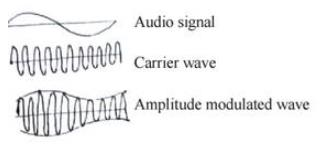Q 39. If a, b, c, d are inputs to a gate and x is its output, then as per the following time graph, the gate is:    
(1) NOT
(2) AND
(3) OR
(4) NAND
Ans:
(3)
Solution:
x is 1 when at least one of the inputs is 1.
Hence x is an OR-Gate.

Q 40. An unknown transistor needs to be identified as npn or pnp type. A multimeter, with +ve and –ve terminals, is used to measure resistance between different terminals transistor. If terminal 2 is the base of the transistor then which of the following is correct for a pnp transistor?    
(1) +ve terminal 3, –ve terminal 2, resistance high
(2) +ve terminal 2, –ve terminal 3, resistance low
(3) +ve terminal 1, –ve terminal 2, resistance high
(4) +ve terminal 2, –ve terminal 1, resistance high
Ans:
(4)
Solution: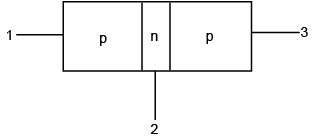when pn junction is forward biased resistance is law. When pn junction is reverse biased, resistance is high

Q 41. An experiment is performed to determine the I - V characteristics of a Zener diode, which has a protective resistance of R= 100 Ω, and maximum dissipation power of rating of 1 Ω. The minimum voltage range of the DC source in the circuit is:    
(1) 0 - 12V
(2) 0 - 5V
(3) 0 - 20V
(4) 0 - 8V
Ans:
(3)
Solution: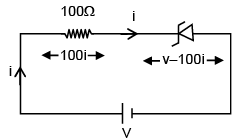Pzener = (v–100i)i = 1
vi – 100i2 =1
100i2 – vi + 1 = 0
i must be real
v2 –4 (100)≥0
v≥20

Q 42. An audio signal consists of two distinct sounds: one a human speech signal in the frequency band of 200 Hz to 2700 Hz, while the other is a high frequency music signal in the frequency band of 10200 Hz to 15200 Hz. The ratio of the AM signal band width required to send both the signals together to the AM signal band width required to send just the human speech is:     
(1) 6
(2) 5
(3) 3
(4) 2
Ans.
(1)
Solution: Band width for both signals    15200 Hz –200 Hz = 15000 Hz
Band width for human speech    2700 Hz – 200 Hz = 2500 Hz
The ratio = 150000/25000 = 6

Q 43. A magnetic dipole is acted upon by two magnetic fields which are inclined to each other at an angle of 75°. One of the fields has magnitude of 15 mT. The dipole attains stable equilibrium at an angle 30° with this field. The magnitude of the other field (in mT) is close to:    
(1) 11
(2) 1060
(3) 36
(4) 1
Ans:
(1)
Solution: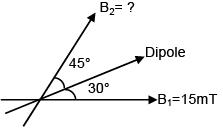B1 sin 30° = B2 sin 45°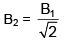= 10.60 mT ≈ 11 mT

Q 44. A modulated signal Cm (t) has the form Cm(t) = 30 sin 300πt + 10 (cos 200πt-cos 400πt). The carrier frequency fc, the modulating frequency (message frequency) fω, and the modulation index μ are respectively given by:    
(1) fc = 200 Hz; fω = 30Hz ; μ = 1/2
(2) fc =  150 Hz; fω = 50Hz ; μ = 2/3
(3) fc = 200 Hz; fω = 50Hz ; μ = 1/2
(4) fc = 150 Hz; fω = 30Hz ; μ = 1/3
Ans:
(2)
Solution:
m(t) = 30 sin (300 πt) + 10 cos (400 πt)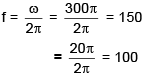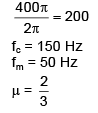Q 45. To get an output of 1 from the circuit shown in figure the input must be: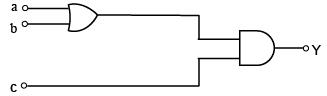(1) a =1, b =1, c = 0
(2) a =1, b =0, c = 0
(3) a =0, b = 0, c = 1
(4) a =1, b =0, c = 1

Ans: (4)
Solution:
Checking options one by one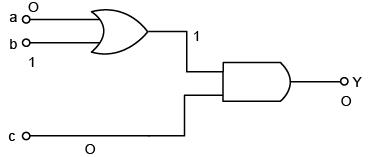We must note that c = 1
and output of OR gate must also be 1
therefore
a =1, b =0, c = 1

Q 46. A realistic graph depicting the variation of the reciprocal of input resistance in an input characteristics measurement in a common emitter transistor configuration is:    
(1)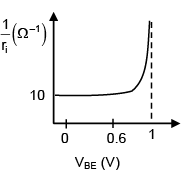(2)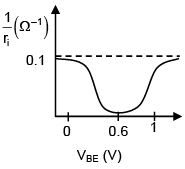(3)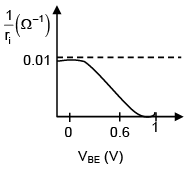(4)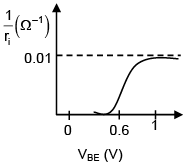Ans: (1)
Solution:

For common emitter configuration, the input characterstics graph is as shown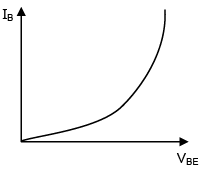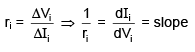Offer running on EduRev: Apply code STAYHOME200 to get INR 200 off on our premium plan EduRev Infinity!

,

,

,

,

,

,

,

,

,

,

,

,

,

,

,

,

,

,

,

,

,

,

,

,

;